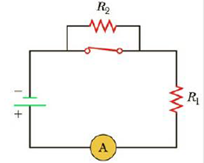Chapter 18.2, Problem 18.3QQ

Chapter
Section
Textbook Problem

In Figure 18.5, the current is measured with the ammeter at the bottom of the circuit. When the switch is opened, does the reading on the ammeter (a) increase, (b) decrease, or (c) not change?Figure 18.5 (Quick Quizzes 18.3 and 18.4)

To determine

The magnitude of current measured in the ammeter.

Explanation

R1, R2 are resistors in the circuit. The current choose to flow from least resistive path. If the resistance of the path increases the flow of current in that path decreases. When the circuit is closed, the current bypass the resistor R2 by flowing through the closed switch and reaches ammeter through the resistor R1 alone.

Conclusion:

When the switch is open now the current cannot bypass the resistor R2

Still sussing out bartleby?

Check out a sample textbook solution.

See a sample solution

The Solution to Your Study Problems

Bartleby provides explanations to thousands of textbook problems written by our experts, many with advanced degrees!

Get Started

Find more solutions based on key concepts